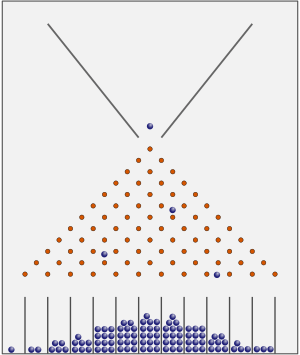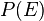# Probability facts for kids

Kids Encyclopedia FactsIn a bean machine or Galton box most balls end up close to the center. In the long run, they will show a normal distribution

Probability is a part of applied mathematics. It has to do with chance, the study of things that might happen or might not happen.

For example, using probability, one can show that by throwing a coin up in the air and letting it land, half of the time it will land with one side facing up, and half of the time with the other side facing up. Many coins have a picture of the face of a famous person on one side, and something else on the other side. Often people call the side with the face "heads", and the other side "tails".

The probability (p) of an event E, written$P(E)$, is always between zero (impossible) and one (certain).

If we roll a die (plural: dice), then the chance that it will land on 1 is 1/6 (because there are 6 numbers on a die). Similarly, the chance it will land on 2 is also 1/6. The chance it will land on any number between 1 and 6 is 1, because every time we roll the die, it will always land on a number between 1 and 6.

Probability can be figured out using mathematics. For example, if one rolls six dice, the chance of them getting a number more than ten is not obvious, but can be figured out using math and science.

One of the most interesting things about chance is that to figure out the probability that two things will both happen, one usually multiply their two probabilities together. For example, suppose that one wants to know the probability of rolling two dice and getting a certain combination (it could be two 6s or a 3 then a 5, just any two). The possibility of getting a 3 is one in six (⅙), and the possibility of getting a 5 is also one in six, so the chances of getting a 3 then a 5 is ⅙×⅙=⅟36. If that number is expressed as somewhere between 0 and 1, it equals 0.027...7, which is fairly low. The possibility of getting a 3, then a 5, and then a 2 would be ⅙×⅙×⅙=⅟216 or 0.00463, which is a much lower probability.

## Ideas of probability

People like Jacob Bernoulli, Pierre-Simon Laplace, or Christiaan Huygens used the word probability, as described above. Other people thought about frequencies; the notion of probability is usually called frequency probability.

## Images for kidsProbability Facts for Kids. Kiddle Encyclopedia.